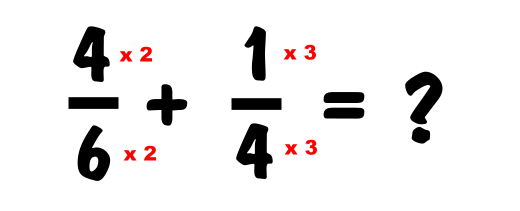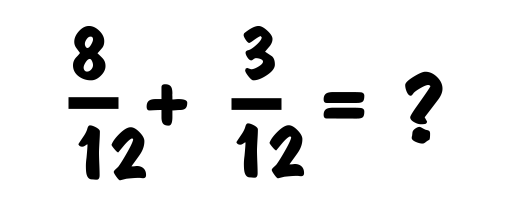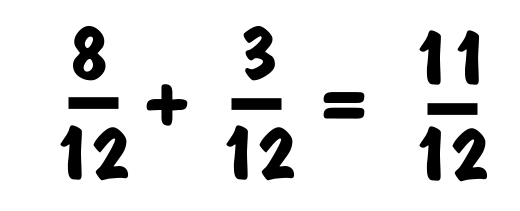How To Add FractionsAdding fractions is pretty easy. We will teach you how to add fractions with this example:To add two fractions, you first need to make the the denominators the same. The denominator is the bottom number. To make the denominator the same, you look for the lowest common denominator. The lowest common denominator is the smallest whole number that is divisible by each of the denominators. In this case it is 12. To make both denominators 12, find out what you have to multiply each denominator by to get 12, then use that number to muliply the numerators (top numbers) to keep the fraction intact.After you multiply the above to get the common denominator, then you get the following:Then you simply leave the denominator, but add the numerators together and you have your answer:More information from Research Maniacs: Fraction Calculator The best online fraction calculator. How To Subtract Fractions Learn How To Subtract Fractions here! How To Multiply Fractions Learn How To Multiply Fractions here! How To Divide Fractions Learn How To Divide Fractions here!

Copyright  |   Privacy Policy  |   Social Media  |   Disclaimer  |   Advertise